# Precalculus Assignment - Vectors, Matrices & Determinants

Instructor: John Hamilton

John has tutored algebra and SAT Prep and has a B.A. degree with a major in psychology and a minor in mathematics from Christopher Newport University.

The following homeschool assignment deals with several precalculus concepts, including vectors on the plane, solving linear systems, inverse matrices, and Cramer's rule. The assignment is appropriate for the 12th grade student. Updated: 05/12/2021

## Assignment Explanation and Topic Overview

Are your students determined to learn about determinants? Am I determined to annoy you with more of my lame math jokes? Of course! Anyway, vectors are often used to represent position, while matrices and determinants can be useful for solving linear equations.

In addition to what is mentioned above, this assignment will include augmented matrices.

By the end of this assignment, students will have completed three steps, solved three problems, and turned in an appropriate presentation.

Note: The answers with explanations are situated at the bottom of the page.

## Key Terms

• Augmented matrix: used to solve linear equations
• Higher-order determinants: involve 3 x 3 and larger matrices
• Inverse matrix: multiplies by a given matrix to obtain the identity matrix

### Materials

• Graph paper
• Internet capability
• Paper
• Pencil
• Ruler

Time / Length

• Five days to complete this mathematics assignment
• Ten days to turn in an appropriate presentation

## Assignment Instructions for Students

### Step One

Let's get started! Are you ready to rumble? As you may know, I never get tired of that cool wrestling expression. Anyway, let's perform operations on vectors in the plane. The good news is adding two vectors is pretty much the same as adding two numbers.

Problem # 1:

Add vector A (2, 3) and vector B (5, 6).

### Step Two

Okay, that was pretty easy, so now let's find the dot product of vectors.

Problem # 2:

Find the dot product given both a vector's beginning points and end points.

Vector A is in Quadrant I and moves from (0, 0) to (4, 7).

Vector B is in Quadrant II and moves from (0, 0) to (-8, 9).

### Step Three

#### Part I

We can't take the determinant of a rectangular matrix, but we can take the determinant of a square matrix.

Problem # 3:

Find the determinant of: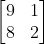#### Part II

Next, let's write an augmented matrix for a linear system:

Example # 1:

x + y = 12

3x - 2y = 16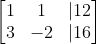That wasn't too difficult, was it? Notice each vertical line represents an equal sign, and take special care that your number 2 has a negative sign.

Example # 2:

Now, let's perform math operations with matrices. If two matrices are the same size, it's pretty easy to add or subtract them, such as: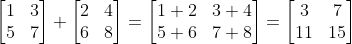Example # 3:

However, multiplying or dividing matrices is a bit more involved: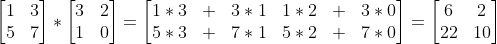#### Part III

We just learned how to solve the determinant for a 2 x 2 matrix, but how do we evaluate higher-order determinants? Take a deep breath! This is a bit challenging.

Your formula for the determinant of a given 3 x 3 matrix is: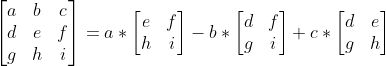Which equals:

a(ei-fh) - b(di-fg) + c(dh-eg)

Whew! I told you to take a deep breath. However, just plug any set of numbers into that formula, and follow the procedure exactly.

#### Part IV

Let's solve a linear system using Gaussian elimination:

Example # 4:

3x + 2y = 7

x - y = -1

3 2 | 7

1 -1 | -1

1 -1 | -1

3 2 | 7

Multiply row 1 by -3 and add the result to row 2:

1 -1 | -1

0 5 | 10

Multiply row 2 by 1/5:

1 -1 | -1

0 1 | 2

We're almost there! What was the whole point of those computations? Did you notice we now have all ones on the diagonal, and a zero in the bottom left corner? Good! These two equations really represent in linear form:

(1)x (-1 )y = (-1)

0x (+1)y = (2)

Or:

x - y = -1

y = 2

x - (2) = -1

x = 1

(1, 2)

Plug (1, 2) back into your original equations:

3x + 2y = 7

x - y = -1

3(1) + 2(2) = 7

(1) - (2) = -1

7 = 7

-1 = -1

Hey, it worked again! We are so good at this precalculus. Let's move on to calculus. Okay, not right now, but maybe next semester.

#### Part V

How do we solve systems of linear equations using determinants? Cramer's rule can be applied.

Let's say you are given this system of linear equations:

Example # 5:

2x + y = 80

x - 3y = -30

Now we utilize Cramer's rule.

Your coefficient determinant D would be: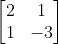= 2 * -3 - 1 * 1

= -6 - 1

= -7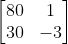See what we did there? We replaced the 2 in your matrix with an 80, and the 1 in your matrix with a -30.

So:

80 * -3 - 1 * -30 =

-240 - -30 =

-210

Now we need to find our Dy determinant. You guessed it! We substitute the constant numbers into the second column.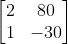So:

2 * -30 - 80 * 1 =

-60 - 80 =

-140

Let's recap:

D = -7

Dx = -210

Dy = -140

We're almost done!

x = Dx / D

y = Dy / D

Therefore:

x = -210 / -7

y = -140 / -7

And:

x = 30

y = 20

To unlock this lesson you must be a Study.com Member.

### Register to view this lesson

Are you a student or a teacher?